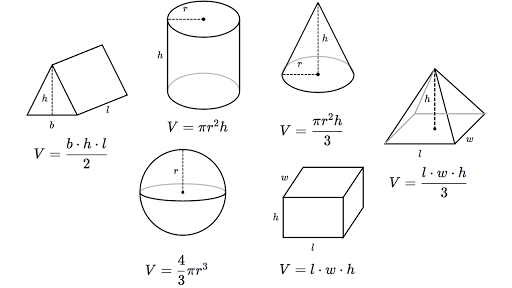# Volume 5/7/19

The past week we learned about solving 3-dimensional shapes for their volume. Volume is the amount of space a 3-dimensional object has which is measured in cubic units. The volume of a circular cone is V=1/3Bh, or V=1/3(pi)r^2h, where B is the area of the base, h is the height of the base, and r is the radius of the base. This formula is used to find the volume of a pyramid. The volume V of a prism is V=Bh, where B is the area of the base and h is the height of the prism. This formula is used to find the volume of a prism. The volume V of a cylinder is V=Bh or V=(pi)r^2h, where B is the area of the base, h is the height of the cylinder, and r is the radius of the base. This formula is used for the volume of a cylinder. These 3-dimensional shape all have different ways to solve them. These shapes are not solved the same because they all have different vertices.